Documentation

### This is machine translation

Mouseover text to see original. Click the button below to return to the English version of the page.

# cp2tform

Infer spatial transformation from control point pairs

`cp2tform` is not recommended. Use `fitgeotrans` instead.

## Syntax

``tform = cp2tform(movingPoints,fixedPoints,transformationType)``
``tform = cp2tform(movingPoints,fixedPoints,'polynomial',degree)``
``tform = cp2tform(movingPoints,fixedPoints,'lwm',n)``
``````tform = cp2tform(movingPoints,fixedPoints,'piecewise linear')``````
``````[tform,usedMP,usedFP,badMP,badFP] = cp2tform(movingPoints,fixedPoints,'piecewise linear')``````
``tform = cp2tform(cpstruct,transformationType,___)``
``````[tform,usedMP,usedFP] = cp2tform(cpstruct,transformationType,___)``````

## Description

example

````tform = cp2tform(movingPoints,fixedPoints,transformationType)` infers a spatial transformation from control point pairs and returns this transformation as a `tform` structure. Some of the transformation types have optional additional parameters, shown in the following syntaxes.```
``` `tform = cp2tform(movingPoints,fixedPoints,'polynomial',degree)` lets you specify the order of the polynomials to use.```
````tform = cp2tform(movingPoints,fixedPoints,'lwm',n)` creates a mapping by inferring a polynomial at each control point using neighboring control points. The mapping at any location depends on a weighted average of these polynomials. You can optionally specify the number of points, `n`, used to infer each polynomial. The `n` closest points are used to infer a polynomial of order 2 for each control point pair.```
``````tform = cp2tform(movingPoints,fixedPoints,'piecewise linear')``` creates a Delaunay triangulation of the fixed control points, and maps corresponding moving control points to the fixed control points. The mapping is linear (affine) for each triangle and continuous across the control points but not continuously differentiable as each triangle has its own mapping.```
``````[tform,usedMP,usedFP,badMP,badFP] = cp2tform(movingPoints,fixedPoints,'piecewise linear')``` returns in `usedMP` and `usedFP` the control points that were used for the piecewise linear transformation. This syntax also returns in `badMP` and `badFP` the control points that were eliminated because they were middle vertices of degenerate fold-over triangles.```
````tform = cp2tform(cpstruct,transformationType,___)` uses a `cpstruct` structure to store the control point coordinates of the moving and fixed images.```
``````[tform,usedMP,usedFP] = cp2tform(cpstruct,transformationType,___)``` also returns in `usedMP` and `usedFP` the control points that were used for the transformation. Unmatched and predicted points are not used. See `cpstruct2pairs`.```

## Examples

### Use Control Points to Create Nonreflective Similarity Transformation Structure

Transform an image, use the `cp2tform` function to return the transformation, and compare the angle and scale of the `tform` to the angle and scale of the original transformation:

```I = checkerboard; J = imrotate(I,30); fixedPoints = [11 11; 41 71]; movingPoints = [14 44; 70 81]; cpselect(J,I,movingPoints,fixedPoints); t = cp2tform(movingPoints,fixedPoints,'nonreflective similarity');```

Recover angle and scale by checking how a unit vector parallel to the x-axis is rotated and stretched.

```u = [0 1]; v = [0 0]; [x, y] = tformfwd(t,u,v); dx = x(2) - x(1); dy = y(2) - y(1); angle = (180/pi) * atan2(dy, dx) scale = 1 / sqrt(dx^2 + dy^2)```

## Input Arguments

collapse all

Control points in the moving image, specified as an m-by-2 matrix. Each row specifies the [x y] coordinates of a control point.

Example: `[11 11; 41 71]`

Data Types: `double`

Control points in the fixed image, specified as an m-by-2 matrix. Each row specifies the [x y] coordinates of a control point.

Example: `[14 44; 70 81]`

Data Types: `double`

Type of transformation, specified as one of the following, listed in order of increasing complexity. The `cp2tform` function requires a minimum number of control point pairs to infer a structure of each transform type.

Transformation Type

Description

Minimum Number of Control Point Pairs

Example

`'nonreflective similarity'`

Use this transformation when shapes in the moving image are unchanged, but the image is distorted by some combination of translation, rotation, and scaling. Straight lines remain straight, and parallel lines are still parallel.

2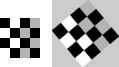`'similarity'`

Same as `'nonreflective similarity'` with the addition of optional reflection.

3`'affine'`

Use this transformation when shapes in the moving image exhibit shearing. Straight lines remain straight, and parallel lines remain parallel, but rectangles become parallelograms.

3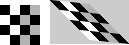`'projective'`

Use this transformation when the scene appears tilted. Straight lines remain straight, but parallel lines converge toward vanishing points that might or might not fall within the image.

4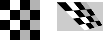`'polynomial'`

Use this transformation when objects in the image are curved. The higher the order of the polynomial, the better the fit, but the result can contain more curves than the fixed image.

You can specify the `degree` of the polynomial.

6 (order 2)

10 (order 3)

15 (order 4)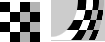`'piecewise linear'`

Use this transformation when parts of the image appear distorted differently.

4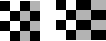`'lwm'`

Use this transformation (local weighted mean), when the distortion varies locally and piecewise linear is not sufficient.

You can specify the number `n` of points to use in the local weighed mean calculation.

6 (12 recommended)Data Types: `char`

Preselected control points, specified as a structure. `cpstruct` contains information about the x- and y-coordinates of all control points in the moving and fixed images, including unpaired and predicted control points. `cpstruct2pairs` eliminates unmatched and predicted control points, and returns the set of valid control point pairs.

`cpstruct` is a structure produced by the Control Point Selection tool (`cpselect`) when you choose the Export Points to Workspace option. For more information, see Export Control Points to the Workspace.

Data Types: `struct`

Degree of the polynomial transformation, specified as the integer `2`, `3`, or `4`.

Data Types: `single` | `double` | `int8` | `int16` | `int32` | `int64` | `uint8` | `uint16` | `uint32` | `uint64`

Number of points to use in local weighted mean calculation, specified as a positive integer. `n` can be as small as 6, but making `n` small risks generating ill-conditioned polynomials

## Output Arguments

collapse all

Transformation, returned as a struct.

Moving control points that were used to infer the spatial transformation, returned as an n-by-2 matrix. Unmatched and predicted points are not used.

Fixed control points that were used to infer the spatial transformation, returned as an n-by-2 matrix. Unmatched and predicted points are not used.

Moving control points that were eliminated because they were determined to be outliers, returned as a p-by-2 matrix.

Fixed control points that were eliminated because they were determined to be outliers, returned as a p-by-2 matrix.

## Tips

• When `transformtype` is `'nonreflective similarity'`, `'similarity'`, `'affine'`, `'projective'`, or `'polynomial'`, and `movingPoints` and `fixedPoints` (or `cpstruct`) have the minimum number of control points needed for a particular transformation, `cp2tform` finds the coefficients exactly.

• If `movingPoints` and `fixedPoints` have more than the minimum number of control points, a least-squares solution is found. See `mldivide`.

• When either `movingPoints` or `fixedPoints` has a large offset with respect to their origin (relative to range of values that it spans), `cp2tform` shifts the points to center their bounding box on the origin before fitting a `tform` structure. This enhances numerical stability and is handled transparently by wrapping the origin-centered `tform` within a custom `tform` that automatically applies and undoes the coordinate shift as needed. As a result, `fields(T)` can give different results for different coordinate inputs, even for the same transformation type.

## Algorithms

collapse all

`cp2tform` uses the following general procedure:

1. Use valid pairs of control points to infer a spatial transformation or an inverse mapping from output space (x,y) to input space (x,y) according to `transformtype`.

2. Return the `tform` structure containing spatial transformation.

The procedure varies depending on the `transformtype`.

### Nonreflective Similarity

Nonreflective similarity transformations can include a rotation, a scaling, and a translation. Shapes and angles are preserved. Parallel lines remain parallel. Straight lines remain straight.

Let

```sc = scale*cos(angle) ss = scale*sin(angle) [u v] = [x y 1] * [ sc -ss ss sc tx ty]```

Solve for `sc`, `ss`, `tx`, and `ty`.

### Similarity

Similarity transformations can include rotation, scaling, translation, and reflection. Shapes and angles are preserved. Parallel lines remain parallel. Straight lines remain straight.

Let

```sc = s*cos(theta) ss = s*sin(theta) [ sc -a*-ss [u v] = [x y 1] * ss a*sc tx ty] ```

Solve for `sc`, `ss`, `tx`, `ty`, and `a`. If `a = -1`, reflection is included in the transformation. If `a = 1`, reflection is not included in the transformation.

### Affine

In an affine transformation, the x and y dimensions can be scaled or sheared independently and there can be a translation. Parallel lines remain parallel. Straight lines remain straight. Nonreflective similarity transformations are a subset of affine transformations.

For an affine transformation,

`[u v] = [x y 1] * Tinv`

`Tinv` is a 3-by-2 matrix. Solve for the six elements of `Tinv`:

`t_affine = cp2tform(movingPoints,fixedPoints,'affine');`

The coefficients of the inverse mapping are stored in `t_affine.tdata.Tinv`.

At least three control-point pairs are needed to solve for the six unknown coefficients.

### Projective

In a projective transformation, quadrilaterals map to quadrilaterals. Straight lines remain straight. Affine transformations are a subset of projective transformations.

For a projective transformation,

`[up vp wp] = [x y w] * Tinv`

where

```u = up/wp v = vp/wp```

`Tinv` is a 3-by-3 matrix.

Assuming

```Tinv = [ A D G; B E H; C F I ]; u = (Ax + By + C)/(Gx + Hy + I) v = (Dx + Ey + F)/(Gx + Hy + I)```

Solve for the nine elements of `Tinv`:

`t_proj = cp2tform(movingPoints,fixedPoints,'projective');`

The coefficients of the inverse mapping are stored in `t_proj.tdata.Tinv`.

At least four control-point pairs are needed to solve for the nine unknown coefficients.

### Note

An affine or projective transformation can also be expressed like this, for a 3-by-2 `Tinv`:

```[u v]' = Tinv' * [x y 1]' ```

Or, like this, for a 3-by-3 `Tinv`:

`[u v 1]' = Tinv' * [x y 1]'`

### Polynomial

In a polynomial transformation, polynomial functions of `x` and `y` determine the mapping.

Second-Order Polynomials

For a second-order polynomial transformation,

`[u v] = [1 x y x*y x^2 y^2] * Tinv`

Both `u` and `v` are second-order polynomials of `x` and `y`. Each second-order polynomial has six terms. To specify all coefficients, `Tinv` has size 6-by-2.

`t_poly_ord2 = cp2tform(movingPoints,fixedPoints,'polynomial');`

The coefficients of the inverse mapping are stored in `t_poly_ord2.tdata`.

At least six control-point pairs are needed to solve for the 12 unknown coefficients.

Third-Order Polynomials

For a third-order polynomial transformation:

`[u v] = [1 x y x*y x^2 y^2 y*x^2 x*y^2 x^3 y^3] * Tinv`

Both `u` and `v` are third-order polynomials of `x` and `y`. Each third-order polynomial has 10 terms. To specify all coefficients, `Tinv` has size 10-by-2.

`t_poly_ord3 = cp2tform(movingPoints, fixedPoints,'polynomial',3);`

The coefficients of the inverse mapping are stored in `t_poly_ord3.tdata`.

At least ten control-point pairs are needed to solve for the 20 unknown coefficients.

Fourth-Order Polynomials

For a fourth-order polynomial transformation:

```[u v] = [1 x y x*y x^2 y^2 y*x^2 x*y^2 x^3 y^3 x^3*y x^2*y^2 x*y^3 x^4 y^4] * Tinv```

Both `u` and `v` are fourth-order polynomials of `x` and `y`. Each fourth-order polynomial has 15 terms. To specify all coefficients, `Tinv` has size 15-by-2.

`t_poly_ord4 = cp2tform(movingPoints, fixedPoints,'polynomial',4);`

The coefficients of the inverse mapping are stored in `t_poly_ord4.tdata`.

At least 15 control-point pairs are needed to solve for the 30 unknown coefficients.

### Piecewise Linear

In a piecewise linear transformation, linear (affine) transformations are applied separately to each triangular region of the image.

1. Find a Delaunay triangulation of the fixed control points.

2. Using the three vertices of each triangle, infer an affine mapping from fixed to moving coordinates.

### Note

At least four control-point pairs are needed. Four pairs result in two triangles with distinct mappings.

### Local Weighted Mean

For each control point in `fixedPoints`:

1. Find the `N` closest control points.

2. Use these `N` points and their corresponding points in `movingPoints` to infer a second-order polynomial.

3. Calculate the radius of influence of this polynomial as the distance from the center control point to the farthest point used to infer the polynomial (using `fixedPoints`).

### Note

At least six control-point pairs are needed to solve for the second-order polynomial. Ill-conditioned polynomials might result if too few pairs are used.

 Goshtasby, Ardeshir, "Piecewise linear mapping functions for image registration," Pattern Recognition, Vol. 19, 1986, pp. 459-466.

 Goshtasby, Ardeshir, "Image registration by local approximation methods," Image and Vision Computing, Vol. 6, 1988, pp. 255-261.